Name:    3.MD.7.D.-Test1B Recognize area as additive.

Multiple Choice
Identify the choice that best completes the statement or answers the question.

1.

What is the area of the following object?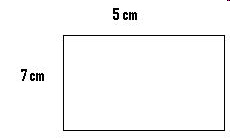a. 12 sq cm c. 35 sq cm b. 24 sq cm d. 27 sq cm

2.

What is the length of the side A?a. 3 cm c. 9 cm b. 6 cm d. 27 cm

3.

What is the length of the side A?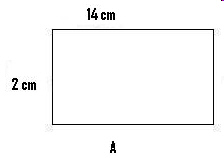a. 13 cm c. 14 cm b. 26 cm d. 16 cm

4.

What is the length of the side A?a. 3 cm c. 2 cm b. 10 cm d. 20 cm

5.

What is the area of the object?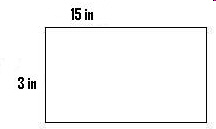a. 24 sq in c. 27 sq in b. 18 sq in d. 45 sq in

6.

What is the area of the object?a. 35 sq in c. 75 sq in b. 120 sq in d. 15 sq in

7.

What is the area of the red section of the rectangle?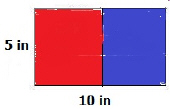a. 15 sq in c. 75 sq in b. 50 sq in d. 25 sq in

8.

What is the length of side A?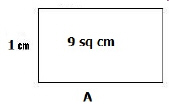a. 20 cm c. 18 cm b. 9 cm d. 11 cm

9.

What is the area of the object?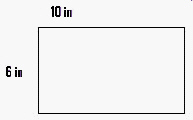a. 32 sq in c. 60 sq in b. 16 sq in d. 26 sq in

10.

To find the area of a rectangle you need to know the length and the _____________.
 a. width c. thickness b. height d. none of these